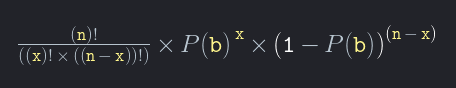“The very name ‘calculus of probabilities’ is a paradox. Probability opposed to certainty is what we do not know, and how can we calculate what we do not know?”
Henry Poincaré

Despite all the talk about probabilities and statistics, it seems that few people can actually calculate mathematically the chance of any given roulette outcome. Sometimes they resort to excel or use specialized programs, trying to test millions of spins in order to come up with the right number. When someone understands basic probability, one can answer almost any question regarding the certainty of any outcome using just a simple calculator or just put the equation as a formula in a simple excel file.First we must understand what is the the factorial function, which has the symbol: !
It means to multiply a series of descending natural numbers.
Examples:
4! = 4 × 3 × 2 × 1 = 24
7! = 7 × 6 × 5 × 4 × 3 × 2 × 1 = 5040
1! = 1
0!=1 (axiomatically)

Practically for roulette purposes, a factorial shows in how many different ways, different items (or numbers) can be arranged. Without repetitions of the same item or number. To give you an idea how huge this number can become, for 37 numbers, like in European roulette:
37! = 1.3763753×1043

This means that there are many trillions of trillions of different arrangements of the 37 roulette numbers. Without counting the possible repetitions of numbers. Just in how many different ways (sequences) all the roulette numbers can appear in 37 spins. You can read more about mathematical combinations here.

## THE PROBABILITY EQUATION

Here is the principal mathematical formula for calculating the chance of any roulette outcome or event.
First we must define the parameters:
P(e) is the probability of an event E.
n is the number of trials (spins)
x is the number of times our bet wins
P(b) is the probability of our bet B winning in one spin

The probability P(e) of the event E = [ Bet b appearing x times in n spins ] =one more time:

### P(e) = (n!/(x!(n-x)!)) P(b)x (1-P(b))n-x

If you want to understand this equation even deeper you can research Binomial distribution which is the foundation of most roulette probabilities. I also want to emphasize the important difference between probability and expectation. Here is a fast and easy method to calculate risk in roulette and this article will help you understand and calculate the expected value of any bet.

Now let’s see how powerful this method is in action. The following examples will help you better understand how the formula works.

## SIMPLE CHANCES EXAMPLE

Let’s say that we want to calculate the probability of two Blacks in three spins. Or to put it differently “how often will we see exactly two Black numbers in three spins”. Note that this equation calculates the exact probabilities of a specific event. Not the probabilities of 2 or more Blacks, but exactly 2 Blacks.
The parameters are:
n = 3 (total spins)
x = 2 (Black numbers/winning spins)
P(b) = 0.5 (the probability of Black in each spin – we ignore the zero for simplicity)

P(e) = (n!/(x!(n-x)!)) P(b)x (1-P(b))n-x
P(e) = (3!/(2!(3-2)!)) 0.52 (1-0.5)3-2
P(e) = (3!/(2!1!)) 0.52 0.51
P(e) = (3×2×1/2×1×1) 0.25× 0.5
P(e) = (3/1) 0.25× 0.5
P(e) = 3 × 0.125 = 0,375

Therefore, the probability in 3 spins to have exactly 2 Black numbers is 0,375 or 37,5% or a little more than 1/3. All these numbers are just different expressions of the same thing – the expectation of the even happening.

## DOZENS EXAMPLE

We want to calculate the probability of a specific (not any) dozen hitting exactly 2 times in 6 spins.
n = 6
x = 2
P(b) = 12/37

P(e) = (6!/(2!(6-2)!)) (12/37)2 (1-12/37)6-2
P(e) = (6×5×4!/(2!4!)) 0.3242 0.6764
P(e) = (30/2) 0.105× 0.209
P(e) = 15 × 0,022
P(e) = 0,329 or 32,9% or around 1/3

## SINGLE NUMBER EXAMPLE

You know that in European roulette, the probability of a specific number appearing in one spin is 1/37 or 2,7%. But what is the probability of a specific number appearing exactly 1 time in 37 spins?The probability of a specific number appearing exactly 1 time in the course of 37 spins is 0.373 or 37,3%.

Using the same formula we can compute the probability of a specific number not appearing at all in 37 spins (0.362 or 36,2%) and the probability of it appearing twice (0.186 or 18,6%).
The mathematical formula we presented here can be applied to find any roulette probabilities in the form of “Bet B hitting X times in N spins”.

### A NUMBER REPEATING 3 TIMES IN 38 SPINS IN AMERICAN ROULETTE

By entering the relevant values into the main equation we get:Which means that the chance of this happening is 0,06 or 6% or 1/16,6.
Consequently, we can expect a phenomena like this to happen once in 633 spins. Since in 38 spins this has probability 1/16,6, we can expect it to occur, on average, after 38*16,6 = 633 spins

Here is a useful calculator where you can COPY and PASTE the EQUATION and then apply the numbers.

Can see more at Khan Academy